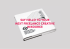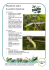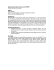Planetary Geology and Atmospheres - Cornell

Transcription

Planetary Geology and Atmospheres - Cornell
Astronomy 1102/1104
Homework 5
Page 1 of 4
Name: ________________________
Section: ________
Spring 2013
Week of March 4
Homework 5: Planetary Geology and Atmospheres
Due in section the week of March 11. The second prelim is on Wednesday, March 13.
Show all work for full credit.
1. Planetary Surface Processes (2.5 points)
(a) Name and describe (in your own words!) the four major geologic processes though
which planetary surfaces evolve.
(b) Fill in the table below.
#
Geological Feature
Ex. Finger Lakes (United States)
1
Chicxulub (Mexico)
2
Cronulla sand dunes (Australia)
3
Grand Canyon (United States)
4
Krakatoa (Indonesia)
5
Mariana Trench (Pacific Ocean)
6
Vredefort Dome (South Africa)
Process
Erosion
Physical Agent
Glaciers
2. Planet Densities (2.5 points)
(a) First, write the equation for a spherical body's average density, in terms of its mass and
radius (and any needed numerical constants). DON'T just say M/V; you should express
V in terms of R.
Astronomy 1102/1104
Homework 5
Page 2 of 4
Now calculate the average density of the following celestial objects, in kilograms per cubic
meter. For comparison, ice is about 1 g/cm3 = 1000 kg/m3, rock is about 3000 kg/m3,
and metal is about 8000 kg/m3. (Hint: You can assume that all of these are objects
spheres. Note that you will first need to express each radius in meters.)
In addition, for each object, compare the calculated density to that of common materials,
such as “Planet X has an average density slightly greater than that of metal” or “Planet
Y is more dense than rock but less dense than metal”.
(b) Earth (mass 5.97×1024 kg, radius 6371 km)
(c) Saturn (mass 5.68×1026 kg, radius 58,232 km)
(d) Titan (Saturn's largest moon – mass 1.35×1023 kg, radius 2575 km)
(e) Kepler-7b (a transiting exoplanet – mass 8.2×1026 kg, radius 103,000 km)
3. Changing Temperatures (2 points)
How would the Earth's average surface temperature change if the following things
happened? That is, would temperatures generally increase or decrease? Explain why.
(Hint: See section 10.2 of the textbook.)
(a) A polar ice cap melts
Astronomy 1102/1104
Homework 5
Page 3 of 4
(b) A polar ice cap expands (so that it covers a larger area)
(c) A large volcanic eruption releases a lot of dust and aerosols into the upper atmosphere
(which decreases the average amount of sunlight reaching the surface)
(d) Earth's orbit shrinks (semimajor axis decreases)
4. Equilibrium Temperature (3 points)
A planet's temperature is determined by the equilibrium between the power it receives (e.g.
from sunlight) and the power it radiates into space. The power it receives from its star is
L
2
P i n=
(1− A)π R p , where L is the star's luminosity (the total power that it
2
4πa
radiates), a is the distance from the star to the planet (the planet's orbital semimajor
axis), A is the planet's albedo (the fraction of incident power that it reflects, so the
fraction it absorbs is (1− A) ), and R p is the planet's radius. Note that this equation
does not account for any internal heat from the planet.
Assuming that the planet is a perfect blackbody, the power it radiates is
4
2
P o u t =(σ T )(4 π R p ) , where the Stefan-Boltzmann constant σ is 5.67×10-8 W/(m2 K4),
and T is the planet's equilibrium temperature. Note that this equation does not
account for radiation that is re-absorbed by the planet's atmosphere.
(a) First, set P i n=P o u t and solve (symbolically) for the equilibrium temperature of the
planet in terms of the other (known) quantities. Don't plug in any numbers yet. (Hint:
You should find that the planet's radius cancels out, so you don't actually need to know
that to find the temperature.)
Astronomy 1102/1104
Homework 5
Page 4 of 4
(b) Calculate the equilibrium temperature of the Earth. The Sun's luminosity is 3.85×1026 W.
Earth's semimajor axis is 1.00 AU = 1.50×1011 m, and its albedo is 0.306. (Hint: Make
sure you're using SI units for everything in this part, and the next parts.)
(c) Compared to the Earth's actual average temperature, is this calculated temperature too
high or too low? What is the most important reason for this? That is, what is the one
physical process that is most responsible for the difference between the temperature
calculated above and the actual temperature?
(d) Calculate the equilibrium temperature of the planet Kepler-22b. Its host star's luminosity
is 79% of the Sun's luminosity. The planet's semimajor axis is 0.85 AU = 1.27×1011 m,
and you can assume for this problem that its albedo is 0.25 (but we don't actually know).

planet dance.pagesGarry Youngberry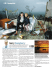Sample Questions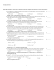The Climate Challenge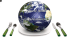The Solar System The Solar System - A to Z Teacher Stuff Printable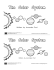Wim Van Gils, Natuurpunt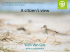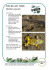Friday 25th October 2013 4.00 p.m. Professor Linda T. Elkins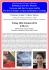to the PDF